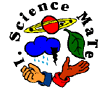Plate Tectonic  SIXTH GRADE Test to review 8 week unit or   take online test (requires Flash) 1. The following picture is of a shield volcano. The arrow is pointing to what structure?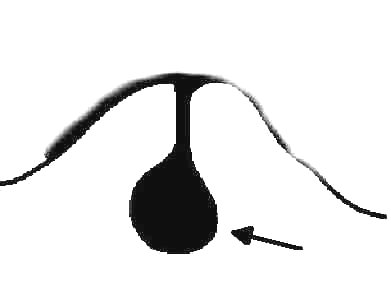A. magma B. summit caldera C. older lava flows D. cinders   2. A composite volcano is unique because it is ________. A. made of lava and ash B. made of lava C. made of ash D. made of cinders 3. The following world map illustrates a unique section of volcanic and earthquake activity. The name of this area is called ________. A. Pacific Ocean B. North America C. Ring of Fire| D. Europe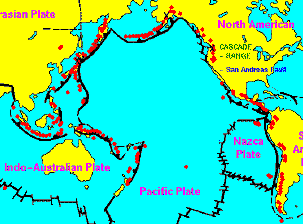4. The elements combined due to volcanic activity to form water are ________. A. silicon and oxygen B. hydrogen and oxygen C. carbon and phosphorus D. oxygen and aluminum 5. You are least likely to find volcanoes at ________. A. converging plate boundaries B. transform C. diverging D. none of these 6. The energy produced by earthquakes travels in ________. A. packets B. wires C. waves D. troughs Examine the diagram below and answer the following questions: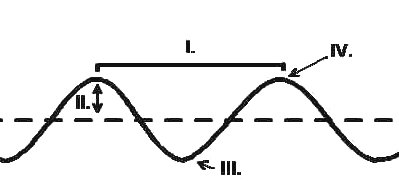7. Where is the wavelength? A. I B. II C. III D. IV 8. Where is the trough? A. I B. II C. III D. IV 9. Where is the waveheight? A. I B. II C. III D. IV 10. Where is the crest? A. I B. II C. III D. IV Use the following diagrams to answer questions 11-13.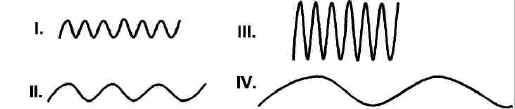11. Which wave represents the greatest energy? A. I B. II C. III D. IV 12. Which has the shortest wavelength? A. I B. II C. III D. IV 13. Which has the longest wavelength? A. I B. II C. III D. IV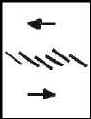14. Identify the following motion at a plate boundary. A. converging B. diverging C. transform D. none of the above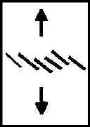15. Identify the following motion at a plate boundary. A. converging B. diverging C. transform D. none of the above16. Identify the following motion at a plate boundary. A. converging B. diverging C. transform D. none of the above ANSWERS: 1.(A); 2.(A); 3.(C); 4.(B); 5.(B); 6.(C); 7.(A); 8.(C); 9.(B); 10.(D); 11.(C); 12.(C); 13.(D); 14.(C); 15.(B); 16.(A)